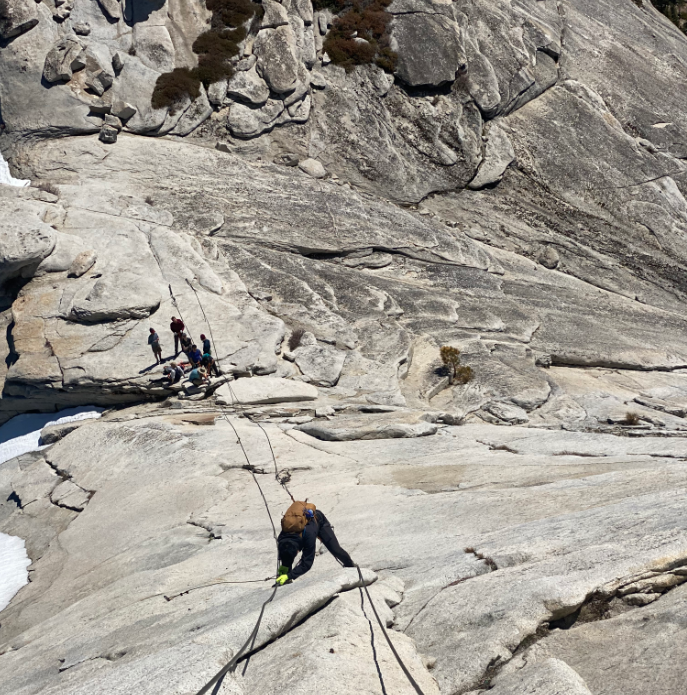## Steel Cables

Consider a 200 ft long steel cable is used to help hikers climb up Half Dome in Yosemite National Park, CA. During the summer, the ambient temperature can reach up to 95°F. If the cable shrinks by a max of 1.2 inches in the winter due to thermal deformation, how cold does Half Dome get in °F? Note the coefficient of thermal expansion of steel is 0.000007 ft/ft°F.Hint
$$\delta_t=\alpha L(T-T_0)$$$where $$\delta_t$$ is the deformation caused by a temp change, $$\alpha$$ is the temp coefficient of expansion, $$L$$ is the member length, $$T$$ is the final temp, and $$T_0$$ is the initial temp. Hint 2 Solve for $$T$$ : $$T=\frac{\delta_t}{\alpha L}+T_0$$$
The equation for thermal deformation is:
$$\delta_t=\alpha L(T-T_0)$$$where $$\delta_t$$ is the deformation caused by a temp change, $$\alpha$$ is the temp coefficient of expansion, $$L$$ is the member length, $$T$$ is the final temp, and $$T_0$$ is the initial temp. Solving for $$T$$ : $$T=\frac{\delta_t}{\alpha L}+T_0$$$
Remember, $$\delta_t$$ is negative because the cables shrink in the winter and to convert the 1.2 inch shrinkage to feet. Thus,
$$T=\frac{-0.1ft(ft^{\circ}F)}{0.000007ft (200ft)}+95^{\circ}F=\frac{-14,285.74^{\circ}F}{200}+95^{\circ}F$$$$$=-71.43^{\circ}F+95^{\circ}F=23.6^{\circ}F$$$
23.6°F